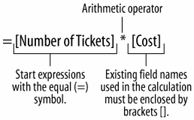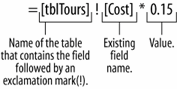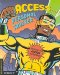# Lesson7.13.Creating a Calculated Control

### Lesson 7.13. Creating a Calculated Control

##### Figure 7-20. To enter database fields in an expression, type the field name in brackets.##### Figure 7-21. If a database field name exists in more than one table, you will need to enter the name of the table that contains the field in brackets, followed by an exclamation mark (!).##### Figure 7-22. You create a calculated field by entering an expression in the Control Source box.A calculated control is an unbound control that displays totals and other arithmetic computations on a form. You create calculated controls by entering an expression (or formula) to perform the calculation in the control's Control Source property.

In forms, expressions start with the equal sign (=), which tells Access that you want to perform a calculation. After the equal sign, you must specify two more types of information: the values you want to calculate and the arithmetic operator(s) or function name(s) you want to use to calculate the values. Expressions can contain explicit values, such as the numbers "4" or "5" or can reference the values contained in database fields. For example, the formula =[Cost]*[Commission] would multiply the values in the Cost and Commissions fields. To enter fields in an expression, type the field name in brackets ([Order Total]), as shown in Figure 7-20. If a field name exists in more than one table, you will need to enter the name of the table that contains the field in brackets ([Customer Tours]) followed by an exclamation mark (!) and then the field, such as [Order Total], as shown in Figure 7-21.

1. Open the frmTours form in Design view.

You want the Total text box to be a calculated control that finds the total cost of each tour by multiplying the Number of Tickets field by the Cost field.

2. Select the Total text box, click the Properties button on the Form Design toolbar, and click the Data tab.

You need to enter the expression in the Control Source box.

3. Click in the Control Source box and type the expression=[Number of Tickets]*[Cost] as shown in Figure 7-22.

Let's see how our new calculated control works.

4. Close the Properties dialog box and click the View button on the Form Design toolbar to switch to Form view.

The Total field displays the results of the expression you entered in Step 3.

5. Click the Close button to close the frmTours form. Click Yes to save.

Although you worked on a form in this lesson, you can also use expressions to create calculated controls and fields in queries and reports.

## QUICK REFERENCE

TO CREATE A CALCULATED CONTROL:

1. DISPLAY THE FORM IN DESIGN VIEW.

2. SELECT THE CONTROL AND CLICK THE PROPERTIES BUTTON ON THE TOOLBAR.

3. CLICK THE DATA TAB AND CLICK IN THE CONTROL SOURCE BOX.

4. TYPE THE EXPRESSION, USING PROPER ACCESS SYNTAX.

OR...

CLICK THE BUILD BUTTON AND USE THE EXPRESSION BUILDER TO CREATE THE EXPRESSION.Access 2003 Personal Trainer (Personal Trainer (OReilly))
ISBN: 0596009372
EAN: 2147483647
Year: 2005
Pages: 209

Similar book on Amazon